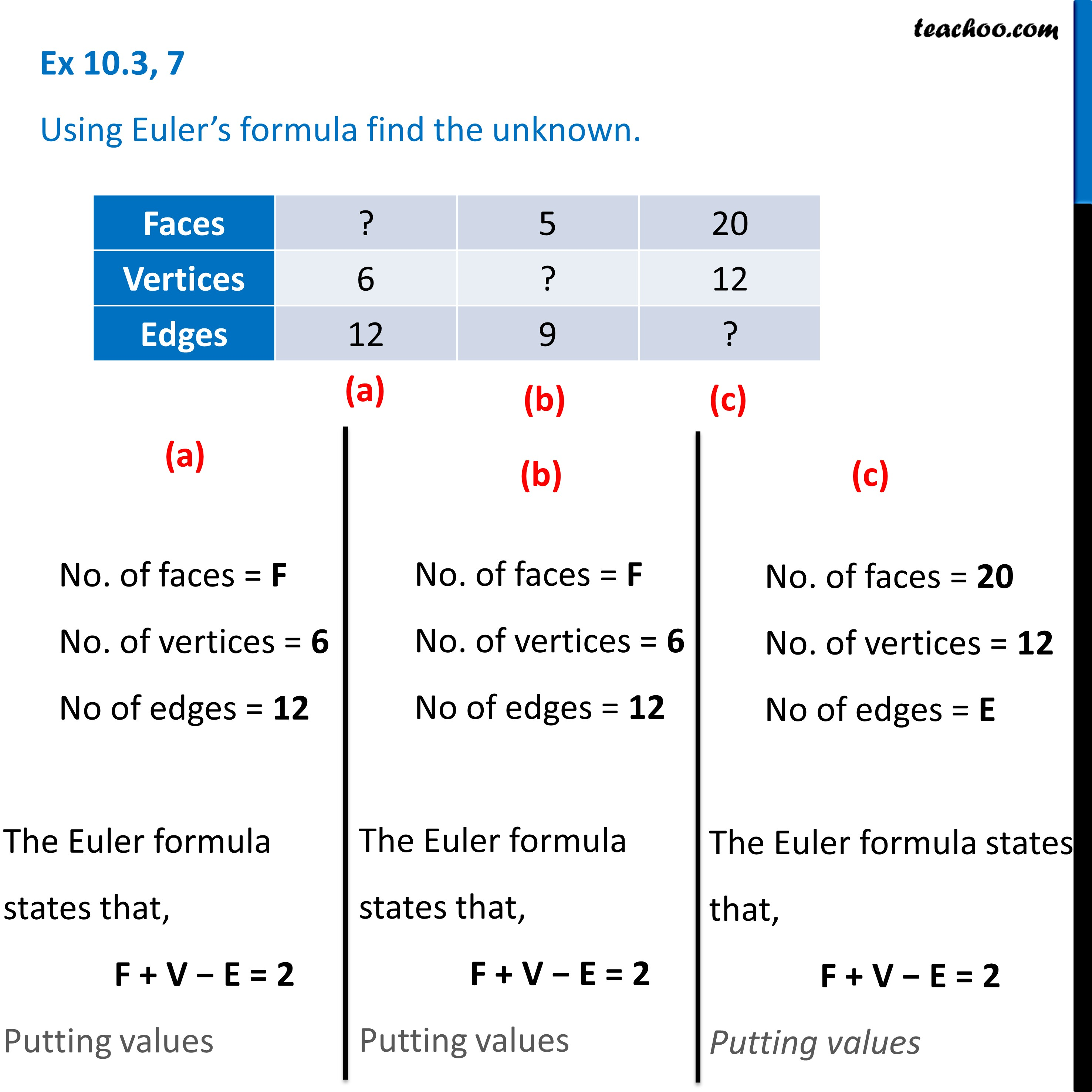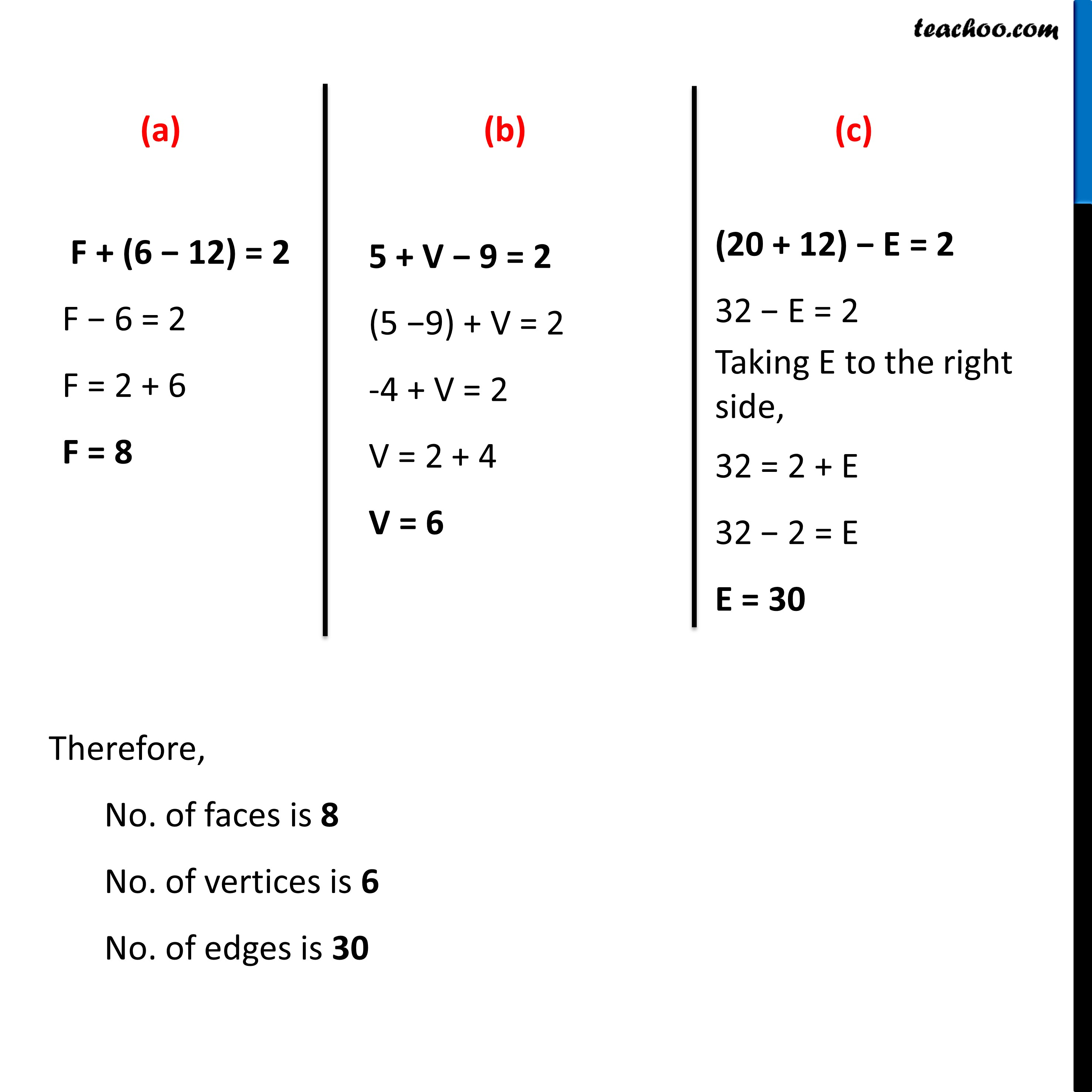Prism and Polyhedrons

Visualising Solid Shapes
Serial order wiseLearn in your speed, with individual attention - Teachoo Maths 1-on-1 Class

### Transcript

Question 7 Using Euler’s formula find the unknown.No. of faces = F No. of vertices = 6 No of edges = 12 The Euler formula states that, F + V − E = 2 Putting values No. of faces = F No. of vertices = 6 No of edges = 12 The Euler formula states that, F + V − E = 2 Putting values No. of faces = 20 No. of vertices = 12 No of edges = E The Euler formula states that, F + V − E = 2 Putting values